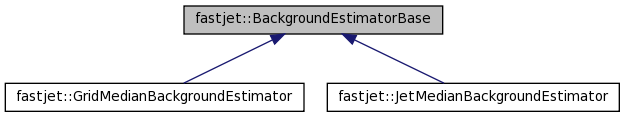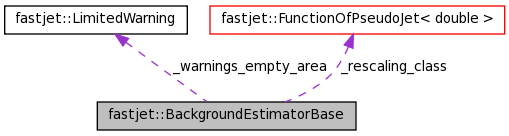FastJet 3.0.4
fastjet::BackgroundEstimatorBase Class Reference

Abstract base class that provides the basic interface for classes that estimate levels of background radiation in hadrion and heavy-ion collider events. More...

#include <fastjet/tools/BackgroundEstimatorBase.hh>

Inheritance diagram for fastjet::BackgroundEstimatorBase:[legend]
Collaboration diagram for fastjet::BackgroundEstimatorBase:[legend]

List of all members.

Public Member Functions

virtual ~BackgroundEstimatorBase ()
a default virtual destructor that does nothing
constructors and destructors
BackgroundEstimatorBase ()
setting a new event
virtual void set_particles (const std::vector< PseudoJet > &particles)=0
tell the background estimator that it has a new event, composed of the specified particles.
retrieving fundamental information
virtual double rho () const =0
get rho, the background density per unit area
virtual double sigma () const
get sigma, the background fluctuations per unit area; must be multipled by sqrt(area) to get fluctuations for a region of a given area.
virtual double rho (const PseudoJet &jet)=0
get rho, the background density per unit area, locally at the position of a given jet.
virtual double sigma (const PseudoJet &)
get sigma, the background fluctuations per unit area, locally at the position of a given jet.
virtual bool has_sigma ()
returns true if this background estimator has support for determination of sigma
configuring the behaviour
virtual void set_rescaling_class (const FunctionOfPseudoJet< double > *rescaling_class_in)
Set a pointer to a class that calculates the rescaling factor as a function of the jet (position).
const FunctionOfPseudoJet
< double > *
rescaling_class () const
return the pointer to the jet density class
description
virtual std::string description () const =0
returns a textual description of the background estimator

Protected Member Functions

helpers for derived classes

Note that these helpers are related to median-based estimation of the background, so there is no guarantee that they will remain in this base class in the long term

void _median_and_stddev (const std::vector< double > &quantity_vector, double n_empty_jets, double &median, double &stand_dev_if_gaussian, bool do_fj2_calculation=false) const
given a quantity in a vector (e.g.
double _percentile (const std::vector< double > &sorted_quantity_vector, const double percentile, const double nempty=0.0, const bool do_fj2_calculation=false) const
computes a percentile of a given _sorted_ vector

Protected Attributes

const FunctionOfPseudoJet
< double > *
_rescaling_class

Static Protected Attributes

static LimitedWarning _warnings_empty_area

Detailed Description

Abstract base class that provides the basic interface for classes that estimate levels of background radiation in hadrion and heavy-ion collider events.

Definition at line 49 of file BackgroundEstimatorBase.hh.

Member Function Documentation

 virtual void fastjet::BackgroundEstimatorBase::set_particles ( const std::vector< PseudoJet > & particles ) [pure virtual]

tell the background estimator that it has a new event, composed of the specified particles.

Implemented in fastjet::GridMedianBackgroundEstimator, and fastjet::JetMedianBackgroundEstimator.

 virtual double fastjet::BackgroundEstimatorBase::sigma ( ) const [inline, virtual]

get sigma, the background fluctuations per unit area; must be multipled by sqrt(area) to get fluctuations for a region of a given area.

Reimplemented in fastjet::GridMedianBackgroundEstimator, and fastjet::JetMedianBackgroundEstimator.

Definition at line 81 of file BackgroundEstimatorBase.hh.

 virtual double fastjet::BackgroundEstimatorBase::rho ( const PseudoJet & jet ) [pure virtual]

get rho, the background density per unit area, locally at the position of a given jet.

Note that this is not const, because a user may then wish to query other aspects of the background that could depend on the position of the jet last used for a rho(jet) determination.

Implemented in fastjet::GridMedianBackgroundEstimator, and fastjet::JetMedianBackgroundEstimator.

 virtual double fastjet::BackgroundEstimatorBase::sigma ( const PseudoJet & ) [inline, virtual]

get sigma, the background fluctuations per unit area, locally at the position of a given jet.

As for rho(jet), it is non-const.

Reimplemented in fastjet::GridMedianBackgroundEstimator, and fastjet::JetMedianBackgroundEstimator.

Definition at line 94 of file BackgroundEstimatorBase.hh.

 virtual void fastjet::BackgroundEstimatorBase::set_rescaling_class ( const FunctionOfPseudoJet< double > * rescaling_class_in ) [inline, virtual]

Set a pointer to a class that calculates the rescaling factor as a function of the jet (position).

Note that the rescaling factor is used both in the determination of the "global" rho (the pt/A of each jet is divided by this factor) and when asking for a local rho (the result is multiplied by this factor).

The BackgroundRescalingYPolynomial class can be used to get a rescaling that depends just on rapidity.

Reimplemented in fastjet::GridMedianBackgroundEstimator, and fastjet::JetMedianBackgroundEstimator.

Definition at line 116 of file BackgroundEstimatorBase.hh.

 void fastjet::BackgroundEstimatorBase::_median_and_stddev ( const std::vector< double > & quantity_vector, double n_empty_jets, double & median, double & stand_dev_if_gaussian, bool do_fj2_calculation = false ) const [protected]

given a quantity in a vector (e.g.

pt_over_area) and knowledge about the number of empty jets, calculate the median and stand_dev_if_gaussian (roughly from the 16th percentile)

If do_fj2_calculation is set to true then this performs FastJet 2.X estimation of the standard deviation, which has a spurious offset in the limit of a small number of jets.

Definition at line 46 of file BackgroundEstimatorBase.cc.

 double fastjet::BackgroundEstimatorBase::_percentile ( const std::vector< double > & sorted_quantity_vector, const double percentile, const double nempty = 0.0, const bool do_fj2_calculation = false ) const [protected]

computes a percentile of a given _sorted_ vector

Parameters:
 sorted_quantity_vector the vector contains the data sample percentile the percentile (defined between 0 and 1) to compute nempty an additional number of 0's (considered at the beginning of the quantity vector) do_fj2_calculation carry out the calculation as it was done in fj2 (suffers from "edge effects")

Definition at line 93 of file BackgroundEstimatorBase.cc.

The documentation for this class was generated from the following files: